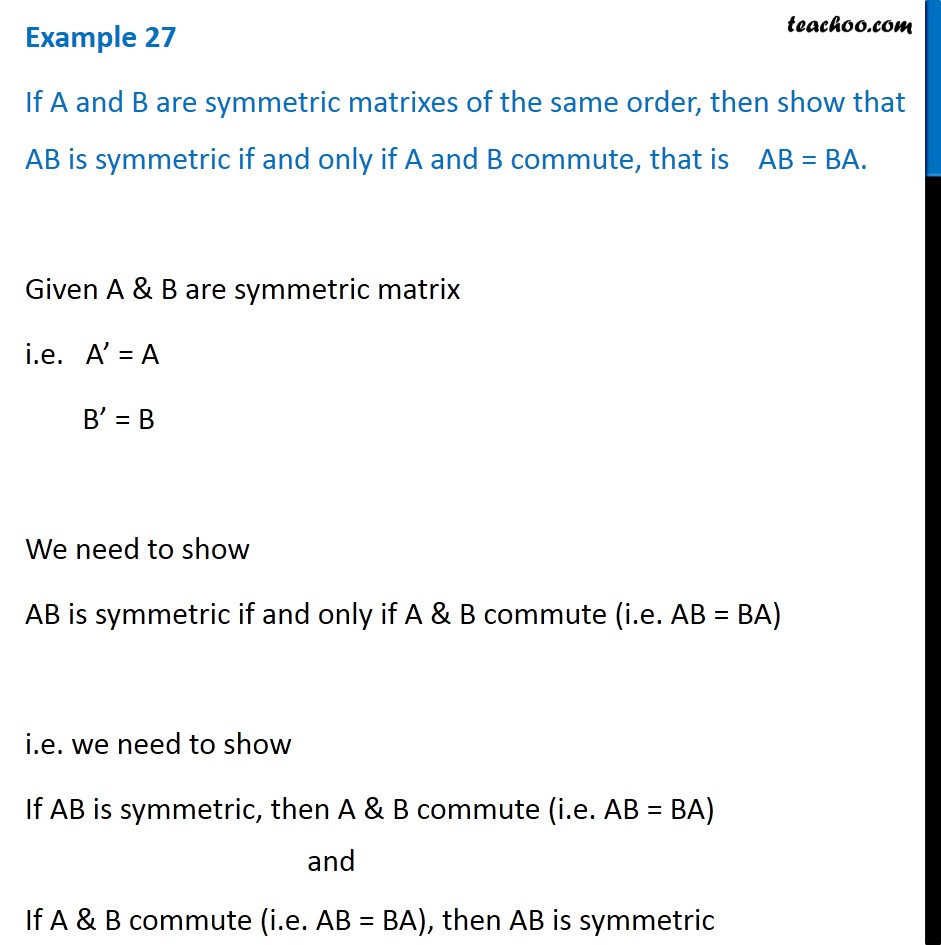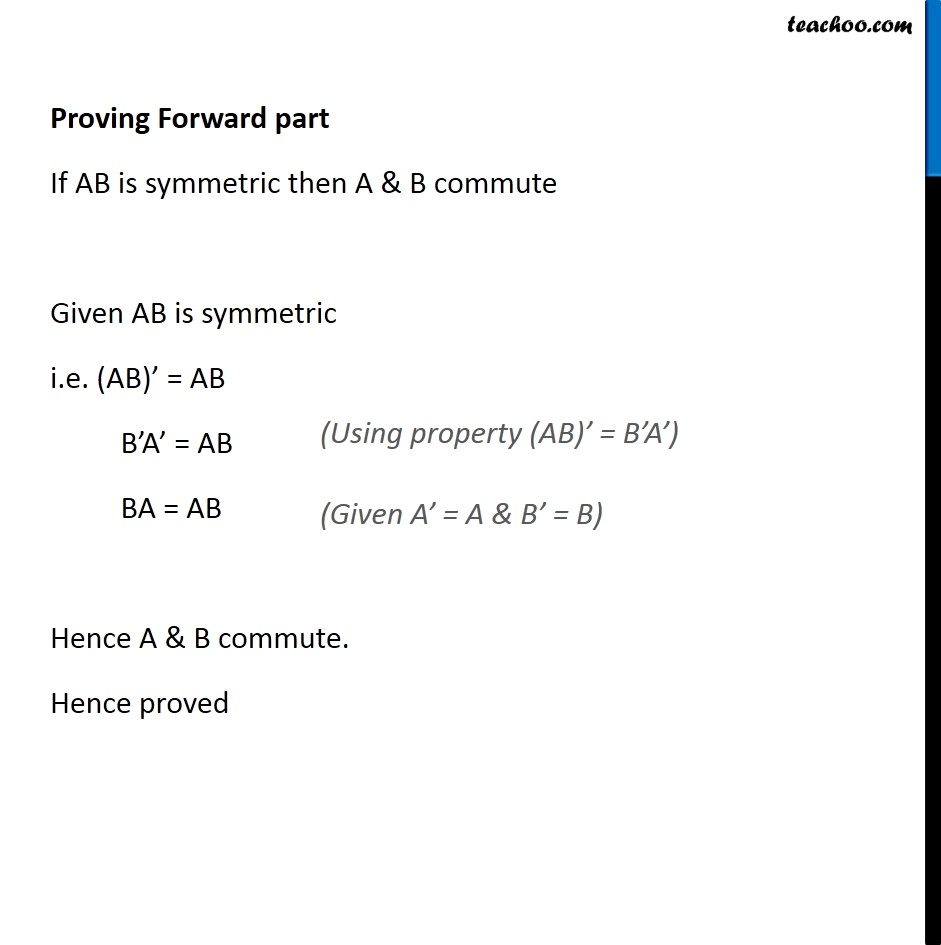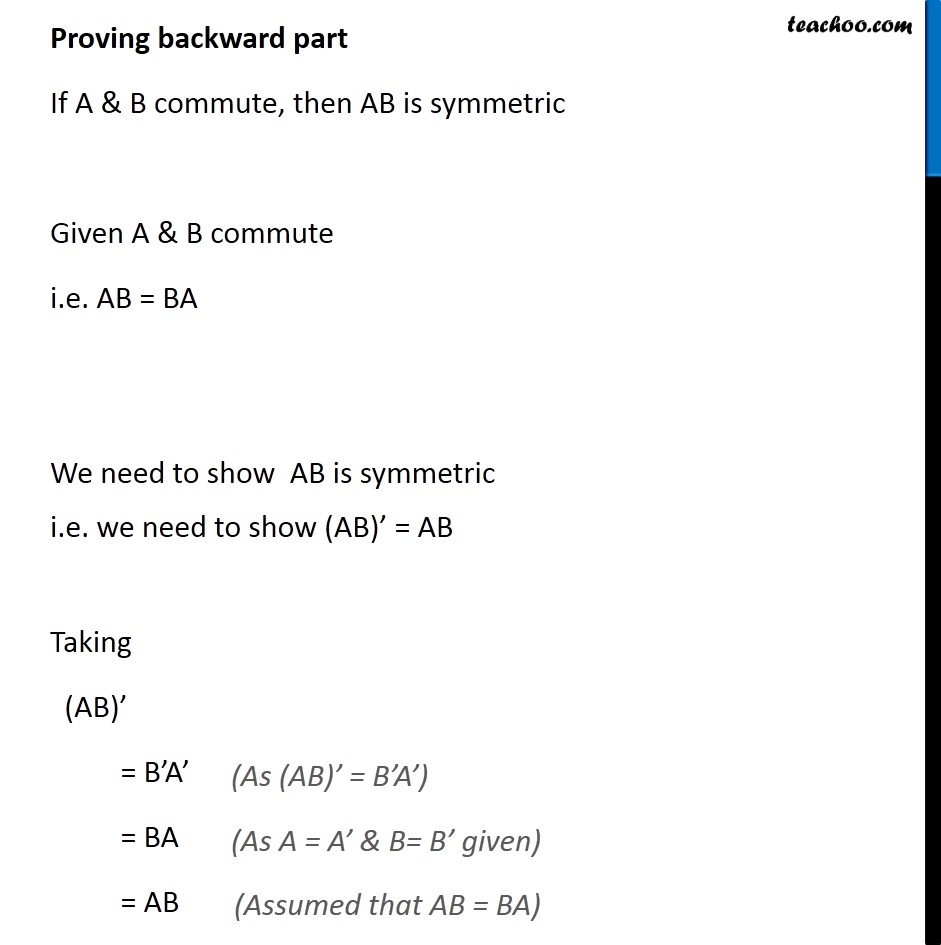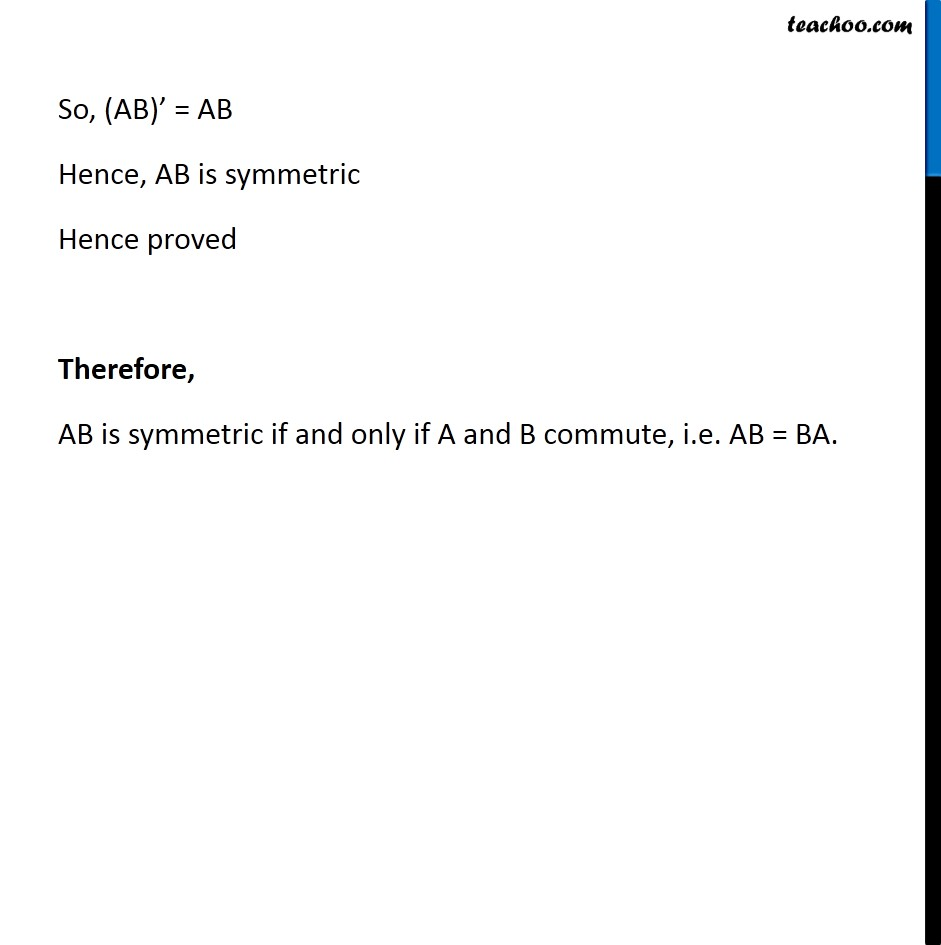Proof using property of transpose

Chapter 3 Class 12 Matrices (Term 1)
Concept wiseNow learn Economics at Teachoo for Class 12

### Transcript

Example 27 If A and B are symmetric matrixes of the same order, then show that AB is symmetric if and only if A and B commute, that is AB = BA. Given A & B are symmetric matrix i.e. A’ = A B’ = B We need to show AB is symmetric if and only if A & B commute (i.e. AB = BA) i.e. we need to show If AB is symmetric, then A & B commute (i.e. AB = BA) and If A & B commute (i.e. AB = BA), then AB is symmetric Proving Forward part If AB is symmetric then A & B commute Given AB is symmetric i.e. (AB)’ = AB B’A’ = AB BA = AB Hence A & B commute. Hence proved (Using property (AB)’ = B’A’) (Given A’ = A & B’ = B) Proving backward part If A & B commute, then AB is symmetric Given A & B commute i.e. AB = BA We need to show AB is symmetric i.e. we need to show (AB)’ = AB Taking (AB)’ = B’A’ = BA = AB (As (AB)’ = B’A’) (As A = A’ & B= B’ given) (Assumed that AB = BA) So, (AB)’ = AB Hence, AB is symmetric Hence proved Therefore, AB is symmetric if and only if A and B commute, i.e. AB = BA.